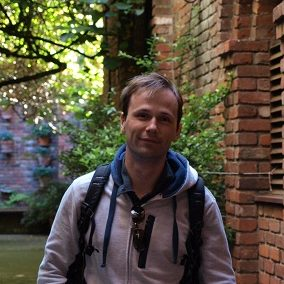Sergiy Neshveyev
(Universitetet i Oslo)
February 16, 2021
15:00
(CET)
Organized by Europe GNCG Seminar.

## Crystallization of C$^*$-algebras

Given a C$^*$-algebra $A$ with an almost periodic time evolution we define a new C$^*$-algebra $A_c$ whose states parameterize the ground states of $A$. We then show, partly by examples, partly by general results, that $A_c$ contains nontrivial information about the equilibrium states on $A$ for low temperatures and its K-theory. (Joint work with Marcelo Laca and Makoto Yamashita.)